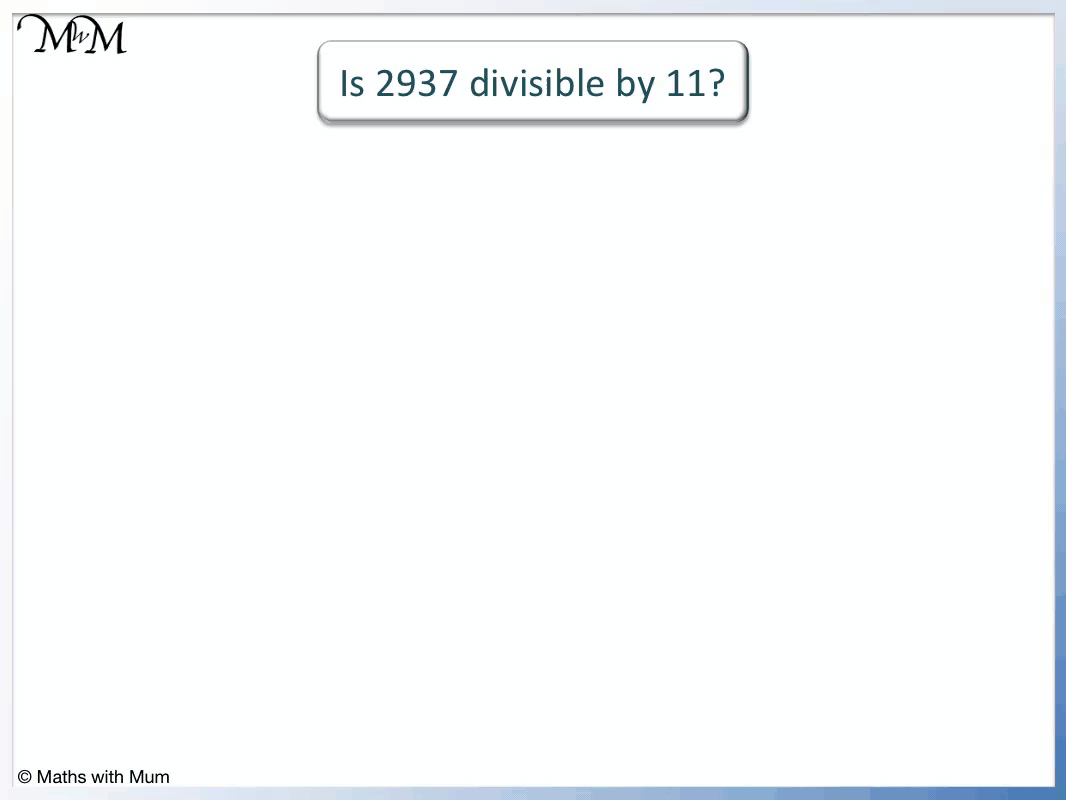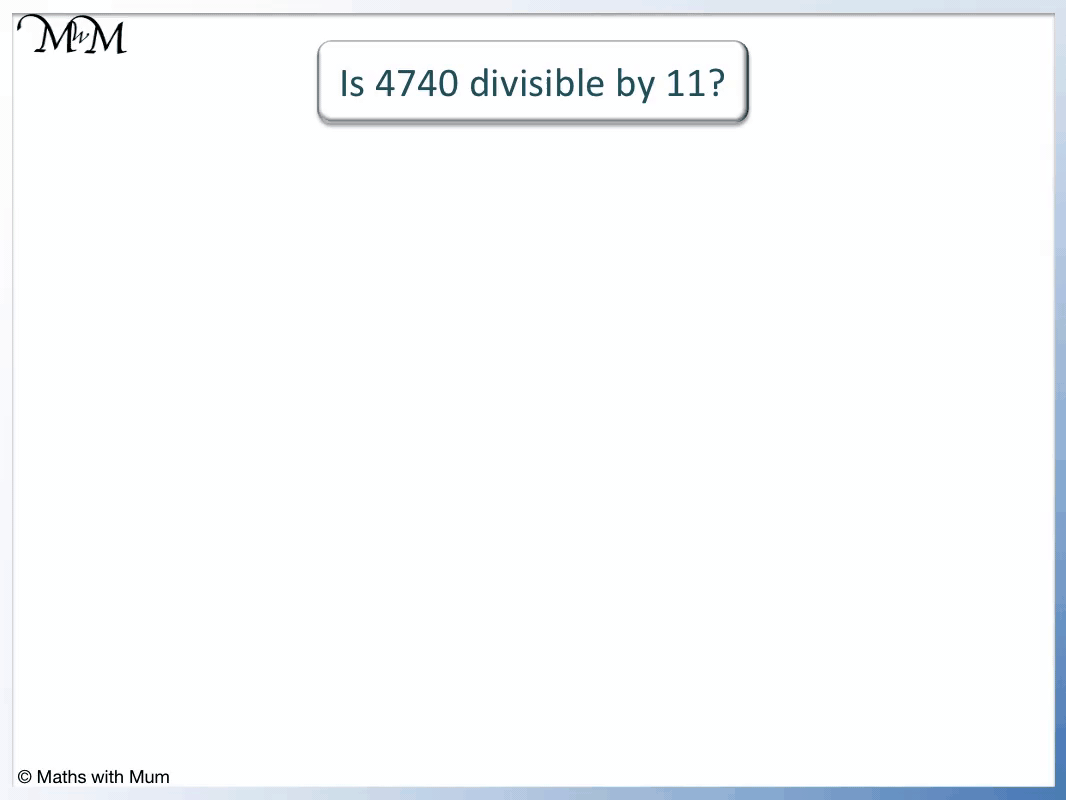# Multiples of 11

Multiples of 11• The multiples of 11 are numbers that can be divided exactly by 11, leaving no remainder.
• The first few multiples of 11 are the numbers in the 11 times table.
• For example 2 × 11 = 22 and so, the second multiple of eleven is 22.
• For example 100 × 11 = 1100 and so, the hundredth multiple of 11 is 1100.

A multiple of 11 is any number that can be divided by 11 exactly, leaving no remainder.#### Multiples of 11 Flashcards

Click on the multiples of 11 flashcards below to memorise the 11 times table:

×
×

=#### Multiples of 11: Interactive Questions

Multiples of 11: Interactive Questions

# Multiples of 11

## What are Multiples of 11

Multiples of 11 are numbers that can be divided exactly by 11. They are formed by multiplying any whole number by 11. For example, the third multiple of 11 is 33 because 3 × 11 = 33.

The first multiples of 11 are shown by the 11 times table. Here is a poster showing the 11 times table.The first 12 multiples of 11 are:

• 1 × 11 = 11
• 2 × 11 = 22
• 3 × 11 = 33
• 4 × 11 = 44
• 5 × 11 = 55
• 6 × 11 = 66
• 7 × 11 = 77
• 8 × 11 = 88
• 9 × 11 = 99
• 10 × 11 = 110
• 11 × 11 = 121
• 12 × 11 = 132

When teaching the multiples of 11, it is useful to show the pattern in the first 9 multiples of 11. The first multiple of 11 are easy to remember because they simply repeat the same digit.

## How to Find Multiples of 11

To find multiples of 11, multiply any whole number by 11. The easiest way to do this is to multiply the number by 10, by writing a zero digit on the end and then adding 1 more lot of the number. For example, the 14th multiple of 11 is 154 because 140 + 14 = 154.

Alternatively the multiples of 11 can be found by starting from 0 and adding on in elevens.

0 + 11 = 11. 11 + 11 = 22. 22 + 11 = 33. 33 + 11 = 44 and so on.

These multiples of 11 are shown below in a number chart. A number chart can be a useful way of teaching the multiples of 11 because we can easily see the diagonal pattern formed by the numbers.We can see that each digit repeats on this chart. This is only true for the first 9 multiples of 11.If we know a multiple of 11, we can easily find the next multiple of 11 by adding on 11.

From 99, we have 99 + 11 = 110 and then 110 + 11 = 121.

We can see that after 99, the multiples of 11 no longer repeat their digits.

The two digit multiples of 11 can be identified by the repeating of their digits. The three digit multiples of 11 can be identified since the first and last digits add up to make the middle digit.

For example, 1 + 1 = 2 in 121, which is a multiple of 11.

## What are the Multiples of 11 up to 100

There are 9 multiples of 11 up to 100. They are:

11, 22, 33, 44, 55, 66, 77, 88 and 99. All of these multiples of 11 contain a repeated digit.

Here are the first multiples of 11 shown on a number chart.Here is a list of the first 100 multiples of 11:

11, 22, 33, 44, 55, 66, 77, 88, 99, 110, 121, 132, 143, 154, 165, 176, 187, 198, 209, 220, 231, 242, 253, 264, 275, 286, 297, 308, 319, 330, 341, 352, 363, 374, 385, 396, 407, 418, 429, 440, 451, 462, 473, 484, 495, 506, 517, 528, 539, 550, 561, 572, 583, 594, 605, 616, 627, 638, 649, 660, 671, 682, 693, 704, 715, 726, 737, 748, 759, 770, 781, 792, 803, 814, 825, 836, 847, 858, 869, 880, 891, 902, 913, 924, 935, 946, 957, 968, 979, 990, 1001, 1012, 1023, 1034, 1045, 1056, 1067, 1078, 1089, 1100.

## The Rule for Identifying Multiples of 11

To decide if a number is a multiple of 11, follow these steps:

1. Starting with the first digit of the number, add every other digit to make a total.
2. Starting with the second digit, add every other digit to make a total.
3. Find the difference between the two totals.
4. If this difference is a multiple of 11 (including 0), the original number is a multiple of 11.
5. If this difference is not a multiple of 11, the original number is not a multiple of 11.

For example, here is 2937. We will use the rule to test if this number is a multiple of 11.

The first digit is 2. We skip the 9 and add the next digit of 3.

2 + 3 = 5.The second digit is 9. We skip the next digit of 3 and add the following digit of 7.

9 + 7 = 16.

The third step is to find the difference between these two totals. We do this by subtracting the smaller number from the larger number.

16 – 5 = 11.

If the difference between the totals is a multiple of 11, the original number is a multiple of 11.

Because the difference is 11, the original number is divisible by 11. Therefore 2937 is a multiple of 11.

Here is an example of a number that is not a multiple of 11. We will use the multiples of 11 rule to prove it.

We have 4740.

The first digit is 4. We skip the 7 and add the next 4.

4 + 4 = 8.The second digit is 7. We skip the next digit of 4 and add the following digit of 0.

7 + 0 = 7.

The third step is to find the difference between the two totals.

8 – 7 = 1.

1 is not a multiple of 11 and so, 4740 is not a multiple of 11 either. This means that 11 does not divide exactly into 4740.

In the next example we can easily see that 495 is a multiple of 11.

Adding alternate digits: 4 + 5 = 9.

The second digit is a 9.Finding the difference, we have 9 – 9 = 0.

0 is a multiple of 11 because 0 × 11 = 0.

Therefore 495 is a multiple of 11.

A 3-digit number is a multiple of 11 if the first and last digits add up to make the middle digit. For example, 495 is a multiple of 11 because 4 + 5 = 9.Now try our lesson on 2-Digit Column Addition where we learn how to use the column addition method to add numbers.